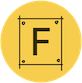#Folk Computer

### Site Tools

folkarc:orbitalkit

# ORBITALKIT

Current issues:

• The speed which the actual circle orbits rotate at are really off

## Source

```# ORBITALKIT - CC0 2023 Arcade Wise
# Create a planet using "Claim \$this is Earth"
# Create a satellite using "Claim \$this is a satellite"

set EarthMass [expr {5.972168 * (10 ** 18)}]
set G [expr {6.67430 * (10.0 ** (-11.0))}]

return [expr {sqrt((\$G * \$EarthMass) / \$radius)}]
}

When /someone/ claims /earth_page/ is Earth {
When \$earth_page has region /earth/ {
# Draw a little earth :>
lassign [region centroid \$earth] x_earth y_earth

Wish to draw a circle with x \$x_earth y \$y_earth radius 100 thickness 5 color green

Wish \$earth_page is labelled Earth

When /someone/ claims /sat_page/ is a satellite  {
When \$sat_page has region /sat/ & the clock time is /t/ & /sat/ has tag /tag/ {
set radius [vec2 distance [region centroid \$earth] [region centroid \$sat]]

# Display the orbit circle
Wish to draw a circle with x \$x_earth y \$y_earth radius \$radius thickness 5 color darkblue filled false

set speed [orbitalVelocityAroundEarth [expr {\$radius * 1000}]]

# Calculate postion for sattelite
set angle [expr {(round((\$t * 200 + (\$tag * 100))) % 2000) / 2000.0}]
set x [expr {(\$radius * sin(6.283 * \$angle )) + \$x_earth}]
set y [expr {(\$radius * cos(6.283 * \$angle )) + \$y_earth}]

# display the stroke
Wish to draw a stroke with points [list [region centroid \$earth] [list \$x \$y]] width 1 color red

Wish to draw a circle with x \$x y \$y radius 20 thickness 5 color white filled true

Wish to draw text with x \$x y [expr {\$y - 40 }] scale 1 text "[expr {round(\$speed)}]km/s at [expr {int(\$radius) * 1000}]km from Earth" radians [region angle \$earth]
}
}
}
}

When tag /tag/ has corners /corners/ & /tag/ has region /region/ {
Claim \$region has tag \$tag
}```